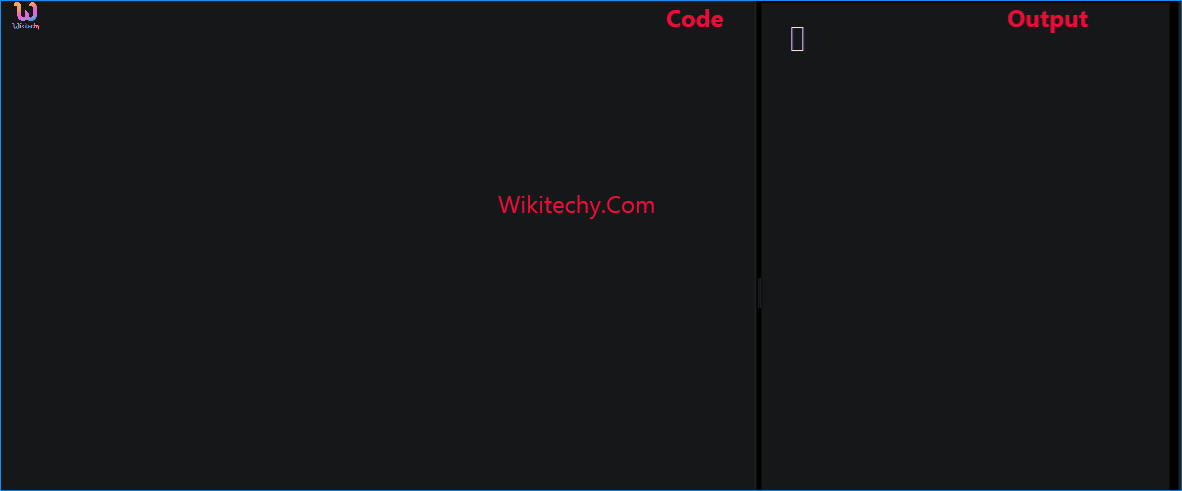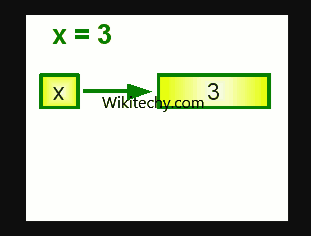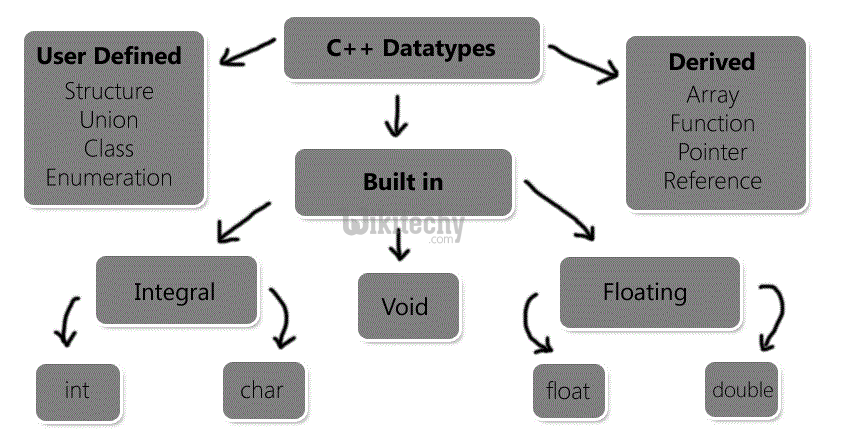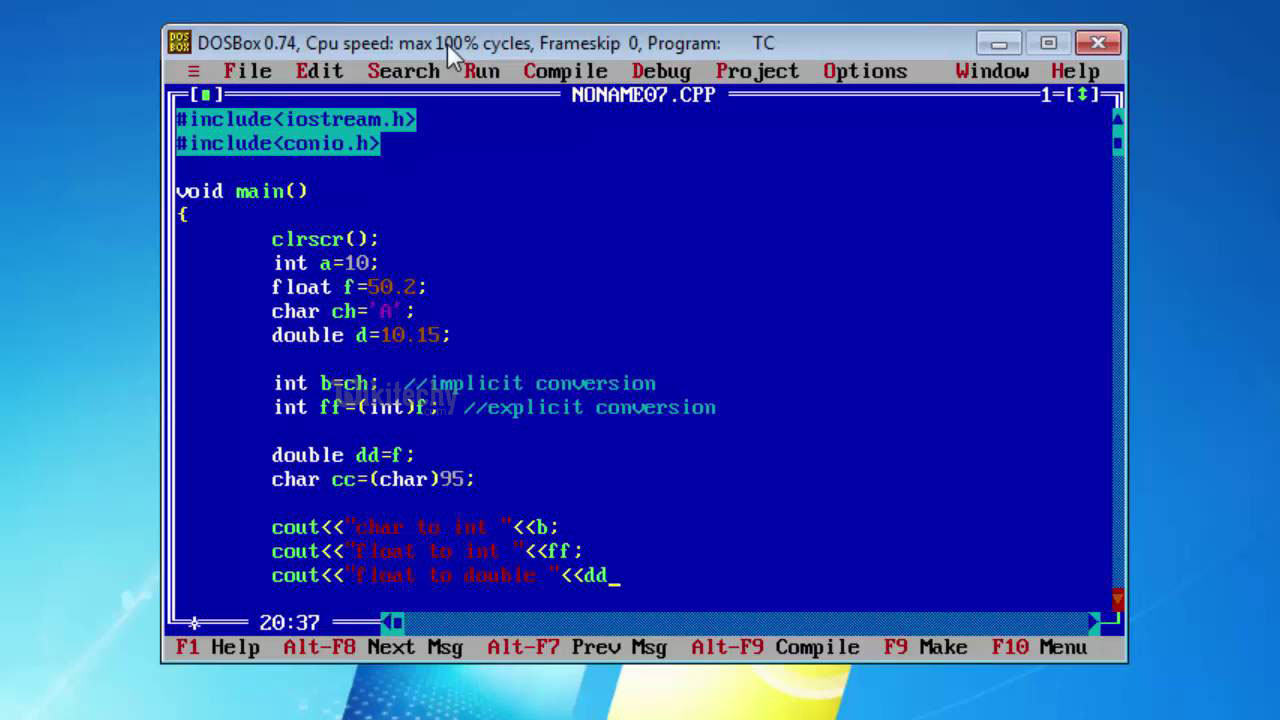# C++ Variables | Data Types and Variables of C++ - Learn C++ , C++ Tutorial , C++ programmingLearn c++ - c++ tutorial - c++ variable - c++ examples - c++ programs

## What is Variables in C++?

• A variable is a name of memory location. It is used to store data. Its value can be changed and it can be reused many times.
• It is a way to represent memory location through symbol so that it can be easily identified.
• It is mandatory to know about the datatypes to assign any variable or to declare a variable.
• While doing programming in any programming language, you need to use various variables to store various information.
•learn c++ tutorials - variables Example

• Variables are nothing but reserved memory locations to store values. This means that when you create a variable you reserve some space in memory.
• You may like to store information of various data types like character, wide character, integer, floating point, double floating point, boolean etc.
• Based on the data type of a variable, the operating system allocates memory and decides what can be stored in the reserved memory.
• A data type specifies the type of data that a variable can store such as integer, floating, character etc.## Primitive Built-in Types

• C++ offer the programmer a rich assortment of built-in as well as user defined data types. Following table lists down seven basic C++ data types:
Type Keyword
Boolean bool
Character char
Integer int
Floating point float
Double floating point double
Valueless void
Wide character wchar_t
• Several of the basic types can be modified using one or more of these type modifiers:
• signed
• unsigned
• short
• long
• The following table shows the variable type, how much memory it takes to store the value in memory what is maximum and minimum value which can be stored in such type of variablesThe sizes of variables might be different from those shown in the above table, depending on the compiler and the computer you are using
Type Typical Bit Width Typical Rangeth
Char 1byte -128 to 127 or 0 to 255
unsigned char 1byte 0 to 255
signed char 1bytes -128 to 127
Int 4byte -2147483648 to 2147483647
unsigned int 4bytes 0 to 4294967295
signed int 4bytes -2147483648 to 2147483647
short int 2bytes -32768 to 32767
unsigned short int 2bytes 0 to 65,535
signed short int 2bytes -32768 to 32767
long int 8bytes -9,223,372,036,854,775,808
to 9,223,372,036,854,775,807
signed long int 8bytes -9,223,372,036,854,775,808
to 9,223,372,036,854,775,807
unsigned long int 8bytes 0 to 18,446,744,073,709,551,615
Float 4bytes +/- 3.4e +/- 38 (~7 digits)
double 8bytes +/- 1.7e +/- 308 (~15 digits)
long double 8bytes +/- 1.7e +/- 308 (~15 digits)
wchar_t 2 or 4 bytes 1 wide character

Following is the example, which will produce correct size of various data types on your computer.

• This example uses endl, which inserts a new-line character after every line and << operator is being used to pass multiple values out to the screen.
• We are also using sizeof() operator to get size of various data types.
• When the above code is compiled and executed, it produces the following result which can vary from machine to machine

## Typedef Declarations:

• You can create a new name for an existing type using typedef. Following is the simple syntax to define a new type using typedef:
• For example, the following tells the compiler that feet is another name for int:
• Now, the following declaration is perfectly legal and creates an integer variable called distance:

## Enumerated Types:

• An enumerated type declares an optional type name and a set of zero or more identifiers that can be used as values of the type.
• Each enumerator is a constant whose type is the enumeration.
• To create an enumeration requires the use of the keyword enum. The general form of an enumeration type is:
• Here, the enum-name is the enumeration's type name.
• The list of names is comma separated.
• For example, the following code defines an enumeration of colors called colors and the variable c of type color.
• Finally, c is assigned the value "blue".
• By default, the value of the first name is 0, the second name has the value 1, the third has the value 2, and so on.
• But you can give a name a specific value by adding an initializer.
• For example, in the following enumeration,green will have the value 5.
• Here, blue will have a value of 6 because each name will be one greater than the one that precedes it.

## Declaring Variable

• Let's see the syntax to declare a variable:
• type variable_list;
• The example of declaring variable is given below:
• int x;
• float y;
• char z;
• Here, x, y, z are variables and int, float, char are data . We can also provide values while declaring the variables as given below:
• int x=5,b=10; //declaring 2 variable of integer type
• float f=30.8;
• char c='A';

## Rules for defining variables

• A variable can have alphabets, digits and underscore.
• No white space is allowed within variable name.
• A variable name must not be any reserved word or keyword e.g. char, float etc.

• int a;
• int _ab;
• int a30;

## Invalid variable names:

• int 4;
• int x y;
• int double;
• A variable in C++ is simply an object that has a name.
• In this c++ tutorial, we are only going to consider integer variables. An integer is a number that can be written without a fractional component
• such as -12, -1, 0, 4, or 27. An integer variable is a variable that holds an integer value.
• In order to create a variable, we generally use a special kind of declaration statement called a definition (we’ll explain the precise difference between a declaration and a definition later).
• Here’s an example of defining variable x as an integer variable (one that can hold integer values):
• When this statement is executed by the CPU, a piece of memory from RAM will be set aside (called instantiation).
• For the sake of example, let’s say that the variable x is assigned memory location 140.
• Whenever the program sees the variable x in an expression or statement, it knows that it should look in memory location 140 to get the value.
• One of the most common operations done with variables is assignment. To do this, we use the assignment operator, more commonly known as the = symbol.
For example: x = 5;
• When the CPU executes this statement, it translates this to “put the value of 5 in memory location 140”.
• Later in our program, we could print that value to the screen using std::cout: